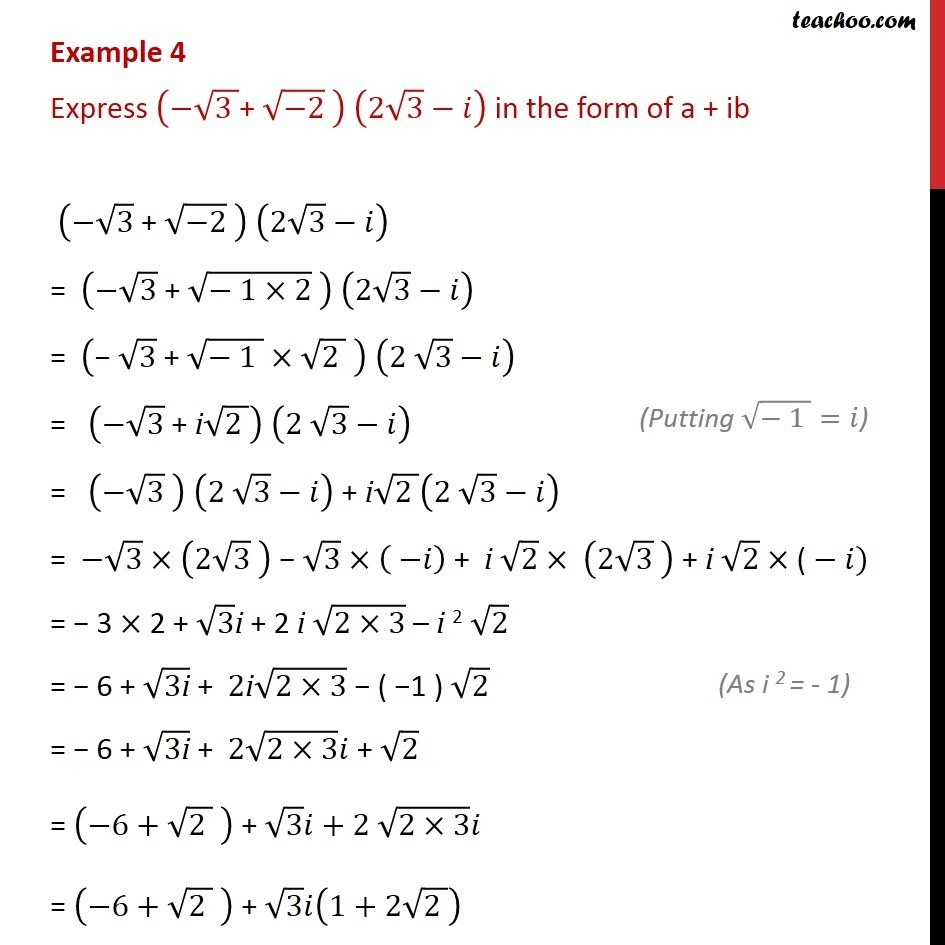Convert to a + ib form

Chapter 4 Class 11 Complex Numbers
Concept wiseLearn in your speed, with individual attention - Teachoo Maths 1-on-1 Class

### Transcript

Example 4 Express ( (3 ) "+ " ( 2) ) (2 3 ) in the form of a + ib ( 3 "+ " ( 2) ) (2 3 ) = ( 3 " + " ( 1 2) ) (2 3 ) = (" " 3 " + " ( 1 ) (2 ) ) (2 3 ) = ( 3 " + i" (2 )) (2 3 ) = ( 3 " " ) (2 3 ) "+ i" (2 ) (2 3 ) = 3 (2 3 ) 3 ( ) + i 2 (2 3 ) + i 2 "(" ) = 3 2 + 3i + 2 i (2 3) i 2 2 = 6 + (3"i" ) + 2"i" (2 3) ( 1 ) 2 = 6 + (3"i" ) + 2 (2 3) + 2 = ( 6+ (2 ) ) + 3 +2 (2 3) = ( 6+ (2 ) ) + 3 (1+2 (2 ))